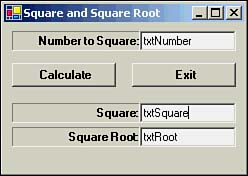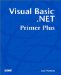# Nested For Loops

### Nested For Loops

We have seen how a For loop can be used to walk through a list of data. In Chapter 7, I showed how multidimensional arrays can be used to process tables of data. Nested For loops provide an easy way to work with tables of data.

To illustrate a nested For loop, let's create a new project named SquaresTable. Place label, text box, and button objects on the form as shown in Figure 12.5.

##### Figure 12.5. Placement of component objects for SquaresTable program.As usual, the Calculate button is named btnCalc and the Exit button is btnExit . The remaining objects are named as shown in Figure 12.5.

Even though the form would suggest that we calculate the square and square root of the number on the fly, this isn't the case. Instead we calculate all possible values as the program is loading, but before presenting the form to the user . This is a common technique used by Visual Basic .NET programmers.

First, we define an array named Values() with module scope. The top several lines of the program are shown in the following code fragment:

` Imports System.Math  Public Class Form1  Inherits System.Windows.Forms.Form  Dim Values(1001, 3) As Double `

The Imports statement for the Math library is necessary because we'll be using the square root routine from the library. The Dim statement defines a Double array with 1001 rows and 3 columns . Listing 12.5 shows how the array elements are processed .

##### Listing 12.5 The Form1_Load Event
` Private Sub Form1_Load(ByVal sender As System.Object, ByVal e As _             System.EventArgs) Handles MyBase.Load  Dim i, j As Integer  For i = 0 To 1000   For j = 0 To 2    Select Case j     Case 0            ' The number      Values(i, j) = i     Case 1            ' Square root      Values(i, j) = Math.Sqrt(i)     Case 2            ' Square      Values(i, j) = i * i    End Select   Next  Next End Sub `

Because we're using two nested loops, we need two loop counter variables , i and j . The outer loop is managed by variable i and the inner loop is managed by j . I've decided to limit the range of numbers from 0 to 1000. The Values() array tracks the value itself, its square, and its square root. (We really don't need the value itself because the array index number is the number being calculated. I just threw the number in to give us an extra number to track.)

We could have used nested If statements to fill in the values, but I decided to use a Select Case statement that uses j to execute the proper statement block. The code in each Case statement is pretty self-explanatory.

All that remains is the btnCalc Click event code, which is shown in Listing 12.6.

##### Listing 12.6 The btnCalc Click Event Code
` Private Sub btnCalc_Click(ByVal sender As System.Object, ByVal e As _              System.EventArgs) Handles btnCalc.Click  Dim Number As Double  If Number > 1000 Then   Beep()   MessageBox.Show("Only values between 1 and 1000")   txtNumber.Focus()  End If  Number = CDbl(txtNumber.Text)  txtSquare.Text = CStr(Values(Number, 2))  txtRoot.Text = Format(Values(Number, 1), "##.#######") End Sub `

First, the code checks to make sure that the number entered by the user falls within the proper range. If the user entered a number that is too large, the computer's alarm is sounded via the call to Beep() and an error message is displayed. After the user reads the message and dismisses the dialog box, control is sent back to txtNumber so that the user can enter a new value.

If the number is within range, Number is assigned the user's input after being converted to a Double . The two text boxes are then filled in with the proper values as held in the Values() array. (Notice how we use Number to index into the array. This is why I said Values(i, 0) is unnecessary.)

That's it! Nested loops are most often used when the dimensions of the data table are known. If you try to use a For loop to index into an array and the loop counter exceeds the upper boundary of the array, you'll get an "out of bounds" error message. If you're unsure of the size of the array, use the UBound() function to set the number of possible passes through the loop.Visual Basic .NET Primer Plus
ISBN: 0672324857
EAN: 2147483647
Year: 2003
Pages: 238
Authors: Jack Purdum

Similar book on Amazon